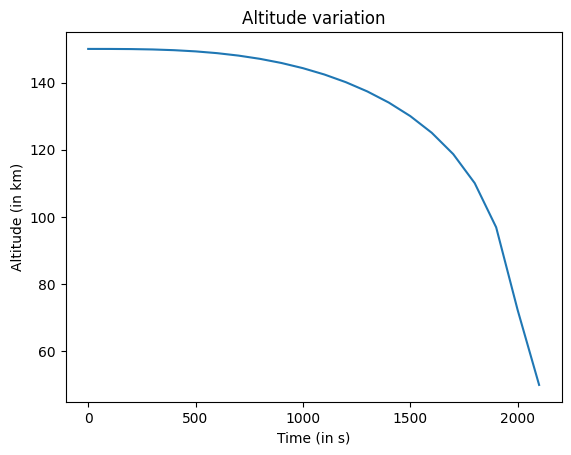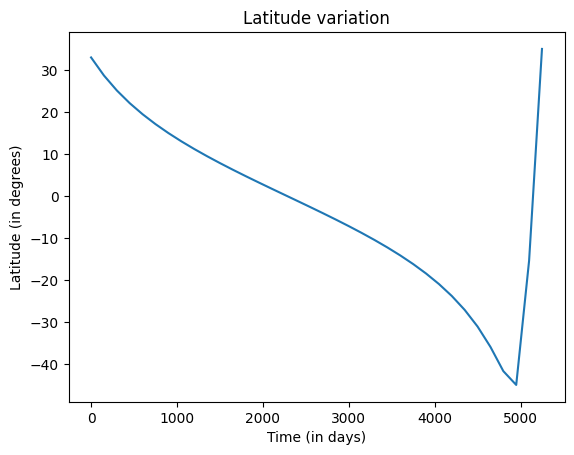# Detecting Events¶

It is a well-known fact that launching a satellite is a captial-intensive and fuel-exhaustive process. Moreover, maintaining high accuracy and precision in any satellite orbit analysis is paramount to be able to comprehend helpful information from them.

Detecing some peculiar phenomena associated with satellites, which we call “events”, could provide beneficial insights about their orbit dynamics for further treatment. While some could provide critical scientific information and help us formulate efficient space strategies and policies, the potentially disastrous ones, like satellite collisions, could help us take further steps to prevent such contingencies.

This notebook gives a glimpse of poliastro’s event detection capabilities. The procedure to track an event during an orbit’s propagation is fairly simple:

1. Instantiate the desired event class/classes.

2. Pass the Event object(s) as an argument to CowellPropagator.

3. Detect events! Optionally, the terminal and direction attributes can be set as required.

:

# Imports
import numpy as np
from numpy.linalg import norm
import matplotlib.pyplot as plt

import astropy
import astropy.units as u
from astropy.time import Time
from astropy.coordinates import (
CartesianRepresentation,
get_body_barycentric_posvel,
)

from poliastro.bodies import Earth, Sun

from poliastro.twobody.events import (
AltitudeCrossEvent,
LatitudeCrossEvent,
NodeCrossEvent,
PenumbraEvent,
UmbraEvent,
)
from poliastro.twobody.orbit import Orbit
from poliastro.twobody.propagation import CowellPropagator
from poliastro.twobody.sampling import EpochsArray

from poliastro.util import time_range


## Altitude Crossing Event¶

Let’s define some natural perturbation conditions for our orbit so that its altitude decreases with time.

:

from poliastro.constants import H0_earth, rho0_earth
from poliastro.core.perturbations import atmospheric_drag_exponential
from poliastro.core.propagation import func_twobody

R = Earth.R.to_value(u.km)

# Parameters of the body
C_D = 2.2  # Dimensionless (any value would do)
A_over_m = ((np.pi / 4.0) * (u.m**2) / (100 * u.kg)).to_value(
u.km**2 / u.kg
)  # km^2/kg

# Parameters of the atmosphere
rho0 = rho0_earth.to_value(u.kg / u.km**3)  # kg/km^3
H0 = H0_earth.to_value(u.km)  # km

def f(t0, u_, k):
du_kep = func_twobody(t0, u_, k)
ax, ay, az = atmospheric_drag_exponential(
t0, u_, k, R=R, C_D=C_D, A_over_m=A_over_m, H0=H0, rho0=rho0
)
du_ad = np.array([0, 0, 0, ax, ay, az])


We shall use the CowellPropagator with the above perturbating conditions and pass the events we want to keep track of, in this case only the AltitudeCrossEvent.

:

tofs = np.arange(0, 2400, 100) << u.s
orbit = Orbit.circular(Earth, 150 * u.km)

# Define a threshold altitude for crossing.
thresh_alt = 50  # in km
altitude_cross_event = AltitudeCrossEvent(thresh_alt, R)  # Set up the event.
events = [altitude_cross_event]

method = CowellPropagator(events=events, f=f)
rr, _ = orbit.to_ephem(
EpochsArray(orbit.epoch + tofs, method=method),
).rv()

print(
f"The threshold altitude was crossed {altitude_cross_event.last_t} after the orbit's epoch."
)

The threshold altitude was crossed 2063.6700936204934 s after the orbit's epoch.


Let’s see how did the orbit’s altitude vary with time:

:

altitudes = np.apply_along_axis(
norm, 1, (rr << u.km).value
) - Earth.R.to_value(u.km)
plt.plot(tofs[: len(rr)].to_value(u.s), altitudes)
plt.title("Altitude variation")
plt.ylabel("Altitude (in km)")
plt.xlabel("Time (in s)")

:

Text(0.5, 0, 'Time (in s)')Refer to the API documentation of the events to check the default values for terminal and direction and change it as required.

## Latitude Crossing Event¶

Similar to the AltitudeCrossEvent, just pass the threshold latitude while instantiating the event.

:

orbit = Orbit.from_classical(
Earth,
6900 << u.km,
0.75 << u.one,
45 << u.deg,
0 << u.deg,
0 << u.deg,
130 << u.deg,
)

:

thresh_lat = 35 << u.deg
latitude_cross_event = LatitudeCrossEvent(orbit, thresh_lat, terminal=True)
events = [latitude_cross_event]

tofs = np.arange(0, 20 * orbit.period.to_value(u.s), 150) << u.s
method = CowellPropagator(events=events)
rr, _ = orbit.to_ephem(EpochsArray(orbit.epoch + tofs, method=method)).rv()
print(
f"The threshold latitude was crossed {latitude_cross_event.last_t} s after the orbit's epoch"
)

The threshold latitude was crossed 5225.7148541757415 s s after the orbit's epoch


Let’s plot the latitude varying with time:

:

from poliastro.core.spheroid_location import cartesian_to_ellipsoidal

latitudes = []
for r in rr:
position_on_body = (r / norm(r)) * Earth.R
_, lat, _ = cartesian_to_ellipsoidal(
Earth.R, Earth.R_polar, *position_on_body
)
plt.plot(tofs[: len(rr)].to_value(u.s), latitudes)
plt.title("Latitude variation")
plt.ylabel("Latitude (in degrees)")
plt.xlabel("Time (in days)")

:

Text(0.5, 0, 'Time (in days)')The orbit’s latitude would not change after the event was detected since we had set terminal=True.

Since the attractor is Earth, we could use GroundtrackPlotter for showing the groundtrack of the orbit on Earth.

:

from poliastro.earth import EarthSatellite
from poliastro.earth.plotting import GroundtrackPlotter
from poliastro.plotting import OrbitPlotter

es = EarthSatellite(orbit, None)

# Show the groundtrack plot from
t_span = time_range(orbit.epoch, end=orbit.epoch + latitude_cross_event.last_t)

# Generate ground track plotting instance.
gp = GroundtrackPlotter()
gp.update_layout(title="Latitude Crossing")

# Plot the above-defined earth satellite.
gp.plot(
es,
t_span,
label="Orbit",
color="red",
marker={
"size": 10,
"symbol": "triangle-right",
"line": {"width": 1, "color": "black"},
},
)